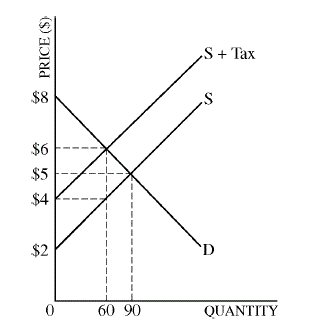2009 Free Response Practice
First Name *
Last Name *
Class Period *The graph above illustrates the market for calculators. S denotes the current supply curve, and D denotes the demand curve.
(a) Calculate the producer surplus before the tax. *(b) Now assume a per-unit tax of \$2 is imposed whose impact is shown in the graph above.
(i) Calculate the amount of tax revenue. *